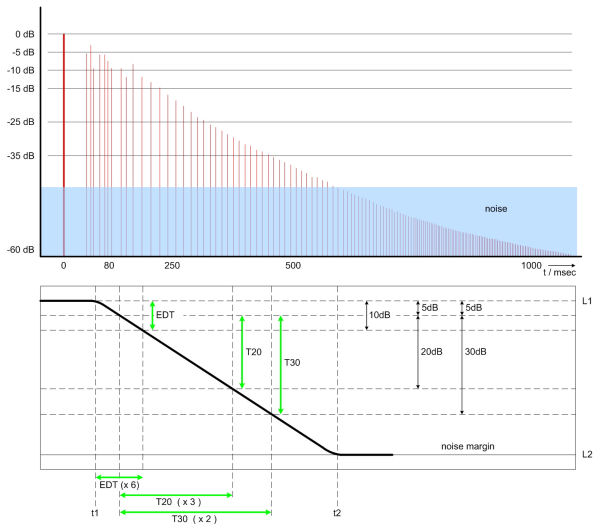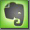Reverberation Time Measurement

Reverberation time is calculated from different measured level ranges:

 Early Decay Time: 0dB to -10dB Reverberation Time T10: -5dB to -15dB Reverberation Time T20: -5dB to -25dB Reverberation Time T30: -5dB to -35dB

To get comparable values all four measurements are multiplied with the appropriate factor to match the historically constituted RT60 (decay of 60 dB).

 Early Decay Time: multiplier 6 Reverberation Time T10: multiplier 6 Reverberation Time T20: multiplier 3 Reverberation Time T30: multiplier 2

Measurements up to 60 dB (the classical RT60) are practically impossible because of always present ambience noise. But the T30 measurement corresponds sufficiently with RT60 and is an adequate result to represent the reverberation time for the full decay.Measurement range for EDT, T10, T20, T30